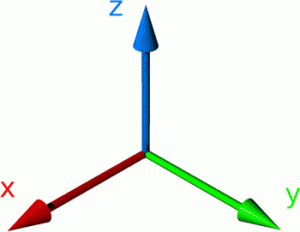# 211. Hidden Rectangle problem

Cool vectors can be exciting! They can describe the motion of a particle, they can represent the acceleration of a rocket, they can tell you about the angle an impact takes place at!Uncool vectors describe lines, they can intersect, they could be perpendicular, they could even describe skew lines in three-dimensions. Not quite as exciting. It isn’t difficult to see that revising standard C4 vectors can be a tad dull. How about an investigation? An investigation without an obvious answer. A question so simple that the answer is a single number. It’s the steps in between that make things interesting…

• I asked my A-Level class to find the area of a rectangle … simple so far, how is this worthy of C4?
• The rectangle is bounded by four vector equations … ok, points of intersection, line segment length, bit of Pythagoras there
• The vector equations are 3D … ooh, that makes it a bit harder
• There are eight equations to choose from … that’s mean, that means finding the angle between lines, checking for skewness, identifying parallel vectors
• There are plenty of ‘red herrings’ … now that is just unfair (great!)

The solution to the problem is a simple surd. If you do ‘Crack the Code’ or ‘Locked Box’ problems you could use the digits under the square root sign as your padlock code.

You can download the worksheet and teachers notes here: C4 Vectors Hidden rectangle (pdf)

Depending on the engagement/ability of the students this could take between 20 and 40 minutes. It would also make an easy to assess homework.

## 3 thoughts on “211. Hidden Rectangle problem”

1.Anita

This is great! I have used this twice in my IB DP Math HL class 🙂

1.admin

Thank you!